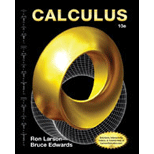# The value of the indefinite integral ∫ x x 2 − 36 d x .### Calculus

10th Edition
Ron Larson + 1 other
Publisher: Cengage Learning
ISBN: 9781285057095### Calculus

10th Edition
Ron Larson + 1 other
Publisher: Cengage Learning
ISBN: 9781285057095

#### Solutions

Chapter 8, Problem 1RE
To determine

## To calculate: The value of the indefinite integral ∫xx2−36dx.

Expert Solution

Solution:

The value of the indefinite integral xx236dx is 13(x236)3/2+C.

### Explanation of Solution

Given:

The integral is xx236dx.

Formula used:

The power formula,

undu=un+1n+1+C

Calculation:

Consider the integral y=xx236dx.

Rewrite the equation as,

y=x236(xdx)

Let u=x236.

Differentiate both sides with respect to x.

dudx=ddx(x236)dudx=ddx(x2)ddx(36)dudx=2x+0du=2xdx

Substitute uforx236 and du2forxdx.

y=u(du2)=12udu=12u1/2du

Solve the above integral as below,

y=12[u12+112+1]+C=12[u32(32)]+C=12[23u32]+C=13u32+C

Resubstitute x236foru.

y=13(x236)3/2+C

Hence, the value of the indefinite integral xx236dx is 13(x236)3/2+C.

### Want to see more full solutions like this?

Subscribe now to access step-by-step solutions to millions of textbook problems written by subject matter experts!

### Want to see more full solutions like this?

Subscribe now to access step-by-step solutions to millions of textbook problems written by subject matter experts!